Robotics and Biomimetics

, 1:10

# Shape of mole nose providing minimum axial resistance

Open Access
Research

## Abstract

### Introduction

As a carrier of different sensors, moles can penetrate into the regolith automatically and keep investigating the subsurface environment continuously. In this section, features of several moles with different applications are introduced to explain why we choose a hammer-driven mole to study.

### Mole driven by a hammer

In this section, the penetrating principle of a hammer-driven mole is illustrated and a circular arc shape for the front nose is proposed. Moreover, applying the penetrating principle, experiments of the mole with an arc-shaped nose are performed to observe the penetration phenomena in a simulated lunar regolith.

### Mechanics analysis

According to soil mechanics theory, regions of soil failure are divided and a mechanics model is established between soil and mole with an arc-shaped nose. The work is done to get approximate axial resistance equations which are analyzed with the defined geometric parameters caliber-radius-head.

### EDEM simulations

EDEM is leading global software based on discrete element method, whose main function is to analyze and observe the movement of particles. Lunar soil simulacrum is established to simulate axial resistance. Eventually, the theoretical results are validated by simulation.

## Keywords

Mole Circular arc shape EDEM

## Background

As a novel technique of in situ investigation, a mole device employs a self-penetrating mechanism, which is considered to be a promising direction for space missions to get information about the geological structure, evolution, and physical and chemical properties of the material if we want to know whether there is life on Mars or the conditions were ever suitable for humans. It is more compact, is lightweight, and has low power consumption. Once it has penetrated into a certain depth, it can acquire geological information of the medium constantly, using various sensors.

In order to conduct deep space exploration, many countries have been involved in the development of a low-speed, unmanned subsurface investigation device. Several prototypes of the mole have been designed and tested in laboratory conditions even though it started late. The mole was first developed by the Russian Federal Space Agency for the Mars-96 mission, called Mars 96 Penetrator, utilizing high speed to penetrate into the regolith, which can penetrate a deeper distance but cannot be used repeatedly and causes great damage to the detecting instruments, leading to data errors . To overcome the disadvantages above, a hammer-driven mechanism was applied to the mole, which will be described in the next sections. In 2011, Japanese researchers proposed a robotic screw explorer which can excavate into soil and transport it backward automatically ,. Inspired by animals, such as mouse and earthworm, more and more researchers focus on the bionics design, e.g., an earthworm-type robot which can make use of the reactive force caused by pushing the discharged regolith above the robot ,. As for China, apart from the development of a thermal drill which is a combination of a rotary drill and a melting probe in Hong Kong Polytechnic University , it is seldom studied so far. In addition, it is worth mentioning that all those prototypes are still in the exploratory pilot phase and none is implemented in space mission successfully, which provides a great space for China to develop the automatic penetrating device.

Although discharging soil backward has great advantage compared with squeezing soil, the hammer-driven mole penetrates better on current technology. So this paper still focuses on optimization of a mole driven by a hammer. As opposed to that of the conventional rotary drill, forward motion of the mole is done by displacement and compression of the soil. Penetration depth depends on the matching of three qualities and shape of the front nose. So it is crucial to study the shape of the front nose. Up to now, the hammer-driven mole has several types of front nose, e.g., MMUM , derived from PLUTO developed by DLR for the Beagle 2 lander on the ESA Mars Express mission with added capability of sampling at the 60° front cone that can open during further penetration ,. Another interesting device is MUPUS on the Philae lander by PAS, whose front nose was designed with sharp and elastic barbs to get anchoring property . Following the successful MUPUS development, a nonlinear conical shape (ogive-shaped tip angle starts from 45° at the base and 30° at the end) was applied to the KRET .

The remainder of this paper is organized as follows. The principle of operation of the hammer-driven mole is illustrated and an arc-shaped front nose is proposed in the ‘Methods’ section. Besides, experiment results of a convex arc-shaped nose in simulated lunar are also presented in the ‘Methods’ section. A mechanical model between the front nose and soil is built in the ‘Results and discussion’ section. What's more, EDEM simulations of the mole with different geometric parameters are given in the ‘Results and discussion’ section to further prove the relationship between axial resistance and caliber-radius-head, followed by the ‘Conclusions’ section.

## Methods

### Principle of penetration

As for the hammer-driven, low-speed type of mole, the basic principle of operation is to make full use of impacting movement to get energy needed for penetration. As shown in Figure 1, the mole mainly consists of seven parts: hammer, servo driving unit, casing, driving spring, buffer spring, escapement mechanism, and tether.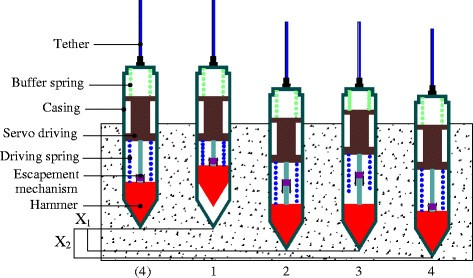Figure 1 Schematic principle of the operation of the mole.
Therefore, the work cycle can be divided into four stages:
1. (a)

Accumulation of energy in the driving spring by the movement of the hammer upward relatively to the casing via the servo driving unit.

2. (b)

Escapement mechanism separates suddenly when the hammer reaches a certain distance and the driven hammer accelerates and hits the bottom of the casing, thus contributing the displacement x 1 by overcoming the restraint of the soil around.

3. (c)

At the instant of the release of the hammer, the servo driving unit moves backward compared with the hammer, and then the buffer spring is compressed to avoid reverse movement of the mole.

4. (d)

Under the effect of the buffer spring and its own gravity, the servo driving unit hits the casing, thus forcing the mole to move down at x 2. The movement of the mole repeats just like that, which is suitable for the regolith that can be compressed for making space. After the mole penetrates to a certain depth, a hole with a higher density appears at the back.

### An arc-shaped front nose

The shape and geometric parameters of the front nose, which contacts soil first, must have a great influence on the penetrating feature of the mole. Consider the axisymmetric mole as a rigid body, remaining undeformed during dynamic penetration. A convex arc shape of the front nose and notations are shown in Figure 2.Figure 2 Cross section of a convex arc-shaped nose and notations.

The shape is arc of a circle with radius S that is tangent to the casing of the mole. Caliber-radius-head is defined as ψ = S/2R, where R is the radius of the casing. The length of the front nose is l.

According to the geometric relationship, the following equations can be obtained:
$\left\{\begin{array}{l}sin{\beta }_{0}=\frac{S-R}{S}\\ l=\sqrt{{S}^{2}-{\left(S-R\right)}^{2}}=R\sqrt{4\psi -1}\end{array}\right\$
(1)

### Penetrating experiments

Automatic penetrating process in space is complicated due to the ultrahigh vacuum, micro-gravity conditions, very low temperature, and huge uncertainties about the surface properties. But in order to further observe the penetration phenomenon of an arc-shaped front nose, penetration experiments have been performed in the laboratory. As shown in Figure 3, experiments have been conducted in the following conditions: (1) target: simulated lunar, (2) caliber-radius-head: 3.7, (3) stroke energy: 1.2 J, (4) mass of the hammer: 1.2 kg, (5) stroke height: 83.3 mm. Facts show that progress of the mole driven by a hammer in a granular medium largely depends on the density of the soil. To ensure the same penetrating conditions, experiments are performed with the same compaction level of simulated lunar.Figure 3 Experiments of the arc-shaped nose.
The arc-shaped nose has been pounded into a 150-mm-diameter and 500-mm-deep barrel containing dry sand which represents a cohesionless material. Recording the penetrating data after ten hammer strokes, each experiment and the result can be seen in Figure 4. The convex arc-shaped nose travels a distance of some 210 mm under 600 strokes in the sand. The performed experiments demonstrate that the nose is capable of good penetration performance in sand. Even though the penetration progress becomes more and more difficult with the increase of depth, the nose is able to move downward under sufficient strokes.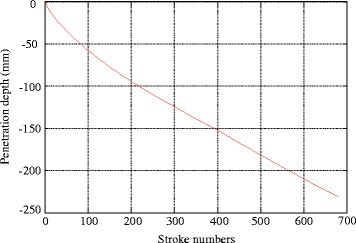Figure 4 Penetration depth vs stroke numbers.

## Results and discussion

### Theory of mechanics analysis

#### Soil failure

As mentioned previously, there is a great deal of complexity about the regolith of space bodies. Currently, it is accepted that the surface and subsurface materials of space bodies consist of granulated matter with grain size in a range from several micrometers to several millimeters, like terrestrial sand . In addition, the mole device is driven by a hammer, which means that it is capable of working in the regolith in the dynamic range. Thus, the mechanical properties and failure model of the regolith are crucial to correctly calculate resistance force. Based on the study of soil on the earth, we assume the mole penetrates a semi-infinite shield.

As shown in Figure 5, soil around the front nose can be divided into three regions called compacted region I, transition region II, and passive region III during the penetration. Due to the effect of large friction on the arc-shaped segments AO and BO, soil in region I moves downward as a rigid body with the mole, and the adjacent region II is forced sideways and region III is displaced upwards. Under positive pressure P, the mole penetrates into the geological layer and soil moves to region III initially. When positive pressure reaches a certain value, soil in region III reaches the limited state. And soil starts to fail if the pressure continues to increase. Region II has two sets of slip lines. One set is two rays, AC from point A and BE from point B. The other set is logarithmic spiral curves CG and EF, expressed as
$r={r}_{0}{e}^{\mathrm{\theta tan\phi }}$
(2)Figure 5 Regions of soil failure around the arc-shaped nose.

where φ is the internal friction angle .

When region III is in the limited state, mechanics analysis of block BEFK is shown in Figure 6. The angle between the slip line and horizontal plane is δ = π/4 − φ/2. After the mole with a convex arc shape penetrates into soil, the initial length of the logarithmic spiral curves is:
${r}_{0}=\overline{\mathrm{BE}}=2\psi R\left(\frac{\sqrt{4\psi -1}}{2\psi -1}sin\beta -cos\beta \right)$
(3)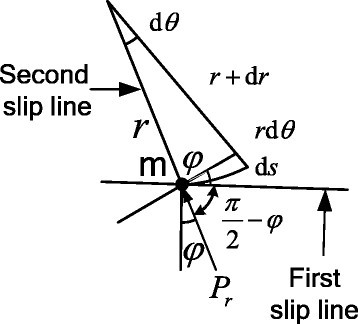Figure 6 Mechanics analysis of block BEFK.
According to geometric relationship, it is easy to see:
$\left\{\begin{array}{l}\overline{\mathrm{B}\mathrm{F}}={r}_{0}{e}^{\left(\frac{\pi }{4}+\frac{\phi }{2}\right)\mathrm{t}\mathrm{a}\mathrm{n}\phi }\\ \overline{\mathrm{B}\mathrm{K}}=\overline{\mathrm{B}\mathrm{F}}cos\delta ={r}_{0}{e}^{\left(\frac{\pi }{4}+\frac{\phi }{2}\right)tan\phi }cos\left(\frac{\pi }{4}-\frac{\phi }{2}\right)\\ \overline{\mathrm{F}\mathrm{K}}=\overline{\mathrm{B}\mathrm{F}}sin\delta ={r}_{0}{e}^{\left(\frac{\pi }{4}+\frac{\phi }{2}\right)tan\phi }\mathrm{sin}\left(\frac{\pi }{4}-\frac{\phi }{2}\right)\end{array}\right\$
(4)
Considering the gravity of BEFK at this time and turning it to pressure acting on the surface of BK:
$q=\gamma H$
(5)
where γ is the bulk density of soil. H is given by
$H=\frac{{r}_{0}}{3}{N}_{\phi }$
(6)
where
${N}_{\phi }=1+\frac{{e}^{\left(\frac{\pi }{2}+\phi \right)tg\phi }{sin}^{2}\left(\frac{\pi }{4}-\frac{\phi }{2}\right)}{1+{e}^{\left(\frac{\pi }{4}+\frac{\phi }{2}\right)tg\phi }sin\left(\frac{\pi }{4}-\frac{\phi }{2}\right)}$
According to the failure criterion of Mohr-Coulomb, the principal stress satisfying the relationship can be expressed as:
${\sigma }_{1}={\sigma }_{3}\mathrm{t}\mathrm{a}{\mathrm{n}}^{2}\left(\frac{\pi }{4}+\frac{\phi }{2}\right)+2ccot\left(\frac{\pi }{4}+\frac{\phi }{2}\right)$
(7)
When soil in region III is in the limited state, longitudinal stress σ z is the minimum principal stress σ3 and horizontal stress σ x is the maximum main stress σ1. So we can get:
${p}_{P}=q\mathrm{c}\mathrm{o}{\mathrm{t}}^{2}\left(\frac{\pi }{4}-\frac{\phi }{2}\right)+2\mathit{c}cot\left(\frac{\pi }{4}-\frac{\phi }{2}\right)$
(8)

where c is cohesion.

Stresses on surface EF are cohesion of the soil c (distribution uniform), normal stress p n , and friction force p n tanφ (distribution non-uniform). p r is the joint stress of p n and p n tanφ, and it has an included angle of φ with p n . According to the characters of the logarithmic spiral curve, point B should be on the force line of p r .

Soil in region I moves downward integrally with the mole. Therefore, BE can be regarded as a retaining wall and the wall back is upright. Suppose the normal stress on surface BE is p F , namely Rankine's active earth pressure. Taking point B as centroid, according to the condition of the static equilibrium of block BEFK, we have:
$\sum {M}_{B}={M}_{{p}_{F}}-{M}_{{p}_{P}}-{M}_{q}-{M}_{c}=0$
(9)
The moment of p r to point B is zero and the rest of the moment to point B are:
$\left\{\begin{array}{l}{M}_{{p}_{F}}=\frac{1}{2}{p}_{F}{\left(\overline{\mathrm{B}\mathrm{F}}\right)}^{2}=\frac{1}{2}{p}_{F}{\left({r}_{0}\right)}^{2}\\ {M}_{{p}_{P}}=\frac{1}{2}{p}_{P}{\left(\overline{\mathrm{H}\mathrm{K}}\right)}^{2}=\frac{1}{2}{\left({r}_{0}\right)}^{2}{e}^{\left(\frac{\pi }{2}+\phi \right)\mathrm{t}\mathrm{a}\mathrm{n}\phi }\left[\gamma H{cos}^{2}\left(\frac{\pi }{4}-\frac{\phi }{2}\right)+ccos\phi \right]\\ {M}_{q}=\frac{1}{2}q{\left(\overline{\mathrm{B}\mathrm{K}}\right)}^{2}=\frac{1}{2}\gamma H{\left({r}_{0}\right)}^{2}{e}^{\left(\frac{\pi }{2}+\phi \right)\mathrm{t}\mathrm{a}\mathrm{n}\phi }{cos}^{2}\left(\frac{\pi }{4}-\frac{\phi }{2}\right)\\ {M}_{c}=\int c\mathrm{d}scos\phi r={\int }_{0}^{\left(\frac{\pi }{4}+\frac{\phi }{2}\right)}c\frac{r\mathrm{d}\theta }{cos\phi }cot\phi r=\frac{1}{2}c{\left({r}_{0}\right)}^{2}cot\mathit{\phi }\left[{e}^{\left(\frac{\pi }{2}+\phi \right)tan\phi }-1\right]\end{array}\right\$
(10)
Substituting Equation 10 into Equation 9, we can get
${p}_{P}=q{N}_{q}+c{N}_{c}$
(11)
where
${N}_{q}=2{e}^{\left(\frac{\pi }{2}+\phi \right)tan\phi }{cos}^{2}\left(\frac{\pi }{4}-\frac{\phi }{2}\right)$
${N}_{c}=\left(cos\phi +cot\mathit{\phi }\right){e}^{\left(\frac{\pi }{2}+\phi \right)tan\phi }-cot\phi$

N q and N c are the bearing capacity coefficients of soil, which are functions of internal friction angle φ.

Mechanics analysis of block BOE in the compacted region is shown in Figure 7, neglecting the gravity of the soil. Increments of horizontal force and vertical force are:
$\left\{\begin{array}{l}\mathrm{d}{Q}_{x}=\left({p}^{\prime }sin\theta -{f}^{\prime }cos\theta \right)\mathrm{d}s\\ \mathrm{d}{Q}_{y}=\left({p}^{\prime }sin\theta +{f}^{\prime }cos\theta \right)\mathrm{d}s\end{array}\right\$
(12)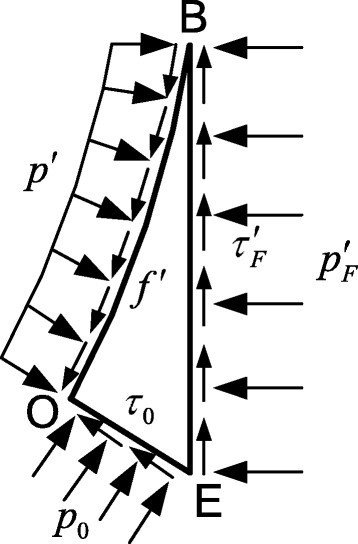Figure 7 Mechanics analysis of block BOE.
where ds is the increment of the arc length on the surface of the nose and p′ is the radial stress acting on the cambered surface BO. Take the coefficient of friction to be μ and
${f}^{\prime }=\mu {p}^{\prime }$
(13)
Substituting Equation 13 into Equation 12 and integraling, horizontal force and vertical force on the cambered surface BO can be expressed as follows:
$\left\{\begin{array}{l}{Q}_{x}=S{p}^{\prime }\left[\frac{\sqrt{4\psi -1}+\mu \left(2\psi -1\right)}{2\psi }-cos\beta -\mu sin\beta \right]\\ {Q}_{y}=S{p}^{\prime }\left[\frac{\mu \sqrt{4\psi -1}-\left(2\psi -1\right)}{2\psi }+sin\beta -\mu cos\beta \right]\end{array}\right\$
(14)
According to the condition of the static equilibrium of block BOE, we find:
$\left\{\begin{array}{l}{Q}_{x}+{p}_{0}cos{\beta }_{0}\overline{\mathrm{O}\mathrm{E}}-{\tau }_{0}sin{\beta }_{0}\overline{\mathrm{O}\mathrm{E}}-{p}_{F}^{\prime }\overline{\mathrm{BE}}=0\\ {p}_{0}sin{\beta }_{0}\overline{\mathrm{O}\mathrm{E}}+{\tau }_{0}cos{\beta }_{0}\overline{\mathrm{O}\mathrm{E}}+{\tau }_{F}^{\prime }\overline{\mathrm{BE}}-{Q}_{y}=0\end{array}\right\$
(15)
where τ0 and ${\tau }_{F}^{\prime }$ can be expressed as:
$\left\{\begin{array}{l}{\tau }_{0}=c+{p}_{0}tan\phi \\ {\tau }_{F}^{\prime }=c+{p}_{F}^{\prime }tan\phi \end{array}\right\$
(16)
Considering the interaction with block BEFK, we can find:
${p}_{F}={p}_{F}^{\prime }$
(17)
Combining Equation 14 to Equation 17, radial stress on surface BO is obtained:
${p}^{\prime }=a\psi +b$
(18)
where
$a=\frac{2\gamma R{N}_{\phi }{N}_{q}\left(1-{tan}^{2}\phi \right){sin}^{2}\left(\beta -{\beta }_{0}\right)}{3sin{\beta }_{0}e}$
$b=c\frac{sin{\beta }_{0}sin\left(\beta -{\beta }_{0}\right)\left({N}_{c}-tan\phi -cot{\beta }_{0}\right)-cos2{\beta }_{0}\left(sin\beta -\frac{2\psi -1}{2\psi }\right)}{sin{\beta }_{0}e}$
$\begin{array}{l}e=\left(sin2{\beta }_{0}-\mu cos2{\beta }_{0}\right)-\left(\mathit{\mu }tan\mathit{\phi }-1\right)cos{\beta }_{0}cos\beta \\ \phantom{\rule{1.25em}{0ex}}-\mu \left(\mathit{\mu }tan\mathit{\phi }+1\right)sin{\beta }_{0}sin\beta -tan\phi cos\left(\beta -{\beta }_{0}\right)+tan\phi \end{array}$

#### Axial resistance

Assume the mole is always along a vertical direction. Then the penetrating process can be divided into three stages:
$D
During the initial penetration, soil resistance acts on the front nose only. Mechanical analysis of the front nose and infinitesimal circle is shown in Figure 8. Then the infinitesimal force of the infinitesimal area can be expressed as:
$\mathrm{d}F=2\pi x\left(pcos\theta +fsin\theta \right)\mathrm{d}s$
(19)Figure 8 Mechanics analysis of the front nose.

where x = S sin θ − (S − R).

Using a formula from differential geometry, the following expression for axial resistance acting on the convex arc-shaped nose can be derived:
${F}_{1}=\int \mathrm{d}F={\int }_{{\beta }_{0}}^{\beta }2\pi x{p}_{1}S\left(cos\theta +\mu sin\theta \right)\mathrm{d}\theta ={K}_{1}{\psi }^{3}+{K}_{2}\psi$
(20)
where
$\begin{array}{l}{K}_{1}=4\pi {R}^{2}a\left[\mu cos\left({\beta }_{0}+\beta \right)+sin\left({\beta }_{0}+\beta \right)\right]sin\left({\beta }_{0}-\beta \right)\\ \phantom{\rule{1.75em}{0ex}}+4\pi {R}^{2}a\left[2\left(sin{\beta }_{0}-sin\beta \right)-2\mu \left(cos{\beta }_{0}-cos\beta \right)\right]\end{array}$
${K}_{2}=4\pi {R}^{2}b\left[\mu \left(cos{\beta }_{0}-cos\beta \right)+\left(sin\beta -sin{\beta }_{0}\right)+4\mu \left({\beta }_{0}-\beta \right)\right]$
$l
With increase of penetration depth, the front nose penetrates into soil totally. Substituting β = π/2 into Equation 20, we can get the axial resistance ${F}_{1}^{\prime }$ on the total convex arc-shaped front nose. What's more, the lateral surface of the mole also contacts soil, whose mechanical analysis is shown in Figure 9. Regarding the lateral surface as a retaining wall, according to Rankine's earth pressure theory, passive earth pressure pP 1 has the following expression:
${P}_{p}=\gamma \left(D-y\right){K}_{p}+2\mathrm{c}\sqrt{{K}_{P}}$
(21)Figure 9 Mechanics analysis of the lateral surface.

where K P is the passive earth pressure coefficients and K P  = tg2(45° + φ/2).

Therefore, horizontal positive pressure on the infinitesimal surface can be expressed as:
$\mathrm{d}N={p}_{P}\mathrm{d}A=2\pi {p}_{P}R\mathrm{d}y$
(22)
which can be integrated between y = l and (D − l) to give the net positive force N:
$N=\int \mathrm{d}N=\pi {R}^{2}\left[\gamma \frac{{K}_{P}}{R}{\left(D-R\sqrt{4\psi -1}\right)}^{2}+4c\frac{\sqrt{{K}_{P}}}{R}\left(D-R\sqrt{4\psi -1}\right)\right]$
(23)
Then we can get the resistance on the lateral surface:
${F}_{c}=\mu N=\mu \pi {R}^{2}\left[\gamma \frac{{K}_{P}}{R}{\left(D-R\sqrt{4\psi -1}\right)}^{2}+4c\frac{\sqrt{{K}_{P}}}{R}\left(D-R\sqrt{4\psi -1}\right)\right]$
(24)
Combining ${F}_{1}^{\prime }$ on the convex arc-shaped front nose, the axial force resisting motion of the mole can be obtained:
${F}_{1}={{F}_{1}}^{\prime }+{F}_{c}$
(25)
$D>l+L$
When the mole penetrates into the regolith totally, the length of the front nose is smaller than the penetration depth relatively and we can get the region of soil failure in Figure 10.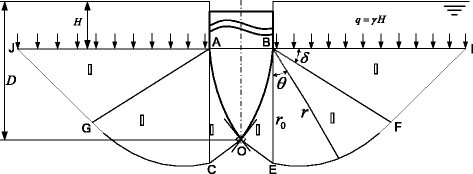Figure 10 Soil failure when the mole penetrates totally.
Neglect the gravity of soil above the front nose and stress on surfaces AJ and BI can be regarded as the gravity of soil above the front nose. Then H is the distance from the ground to surfaces AJ and BI, which can be expressed as:
$H=D-l=D-R\sqrt{4\psi -1}$
(26)
Applying the mechanics analysis of soil block before, we can get the stress on surface BE:
${p}_{F}=\gamma {N}_{q}\left(D-R\sqrt{4\psi -1}\right)+c{N}_{c}$
(27)
Afterwards, radical stress on the contact stress between soil and nose is:
${p}^{″}={a}^{″}\sqrt{4\psi -1}\left(\frac{D}{R}-\sqrt{4\psi -1}\right)+c{b}^{″}$
(28)
where
${a}^{″}=\frac{\gamma R{N}_{q}\left[2tan\phi \frac{\sqrt{4\psi -1}}{2\psi -1}+\left(1-{tan}^{2}\phi \right)\right]}{\left(1-\mathit{\mu }tan\phi \right)\sqrt{4\psi -1}+\left(\mu +tan\phi \right)}$
${b}^{″}=\frac{2\left({N}_{c}tan\phi +1\right)\frac{4\psi -1}{2\psi -1}-\left[tan\phi -{N}_{c}\left(1-{tan}^{2}\phi \right)\right]\sqrt{4\psi -1}-1}{\left(1-\mathit{\mu }tan\phi \right)\sqrt{4\psi -1}+\left(\mu +tan\phi \right)}$
According to Equation 19, integrate dF from θ = β0 and θ = π/2. So axial resistance on the front nose has the following expression:
${F}_{1}^{″}=\pi {R}^{2}\left[{a}^{″}\sqrt{4\psi -1}\left(\frac{D}{R}-\sqrt{4\psi -1}\right)+c{b}^{″}\right]=\left[4\mu \left(\frac{\pi }{2}-{\beta }_{0}\right){\psi }^{2}-\mu \left(2\psi -1\right)\sqrt{4\psi -1}+1\right]$
(29)
Moreover, the lateral surface contacts soil totally in this stage. Combining F c  = μN, integrate Equation 22 between y = l and y = l + L to get the net resistance on the lateral surface:
${F}_{c}^{″}=\mu N={{K}_{1}}^{\prime }-{{K}_{2}}^{\prime }\sqrt{4\psi -1}$
(30)
where
${{K}_{1}}^{\prime }=2\mathit{\mu L}\sqrt{{K}_{P}}\left[\pi R\gamma \sqrt{{K}_{P}}\left(2D-L\right)+c\right]$
${{K}_{2}}^{\prime }=4\pi {R}^{2}\mu \gamma {K}_{P}L$
Then axial resistance of the mole is obtained as follows:
${F}_{1}={{F}_{1}}^{\prime \prime }+{{F}_{c}}^{\prime }$
(31)

Through mechanics analysis, it is known that for a convex arc-shaped front nose, axial resistance is identified with penetration depth and geometric parameters caliber-radius-head. In order to get the relationship between axial resistance and caliber-radius-head directly, other physical parameters which will be used are listed in Table 1 according to the acquaintance of the mole and soil.
Table 1

Physical parameters of the mole and soil

Parameters

R(mm)

L(mm)

c

φ(°)

γ(kN/m3)

μ

Value

15

200

0

30

18

0.3

Based on axial resistance equations, curves relating axial resistance to penetration depth for various values of ψ are drawn (shown in Figure 11). As can be seen from Figure 11, the curve of axial resistance is similar to a parabola changing with penetration depth. Moreover, axial resistance decreases with the increase of ψ. However, the advantage is not obvious when ψ increases to 3 and a very large value of ψ causes an increase in total length of the front nose which is not advisable.Figure 11 Axial resistance vs penetration depth for different ψ .

### EDEM simulation

Generally, granular matter behaves like a compressible non-Newtonian complex fluid including fluid solid transition and can be simulated using EDEM simulation. An obvious advantage of EDEM simulation is that it provides the possibility of obtaining movement, forces, and other dynamical properties of the system at any time.

For ensuring establishment of the simulation model consistent with theoretical analysis, the model of the mole is built in SolidWorks and has the same parameter setting as shown Table 1. Besides, the Hertz-Mindlin (no slip) interaction model is employed in EDEM simulations, and the setting of the other variables is shown in Tables 2 and 3. Simulation of the mole whose shape parameter is 2 is shown in Figure 12 to observe the flow of the lunar soil.
Table 2

Material parameters

Material

Poisson's ratio

Shear modulus (Pa)

Density (kg/m3)

Particle

0.3

2 × 107

1,750

Mole

0.3

2 × 1010

7,850

Table 3

Interaction parameters

Interaction

Particle-particle

Particle-mole

Coefficient of restitution

0.1

0.2

Coefficient of static friction

0.574

0.3

Coefficient of rolling friction

0.01

0.01Figure 12 EDEM simulation of a mole with an arc-shaped front nose (a-c). The shape parameter of the mole is 2(ψ =2), and particles are set with different colors by section to observe the flow directly during the penetration.
It can be seen that the mole works properly in granular matter and the failure of soil increases along with the increase of penetration depth. Change of the values of the shape parameter ψ from 1 to 4 and all simulations are shown in Figure 13.Figure 13 Penetration simulations of mole with an arc-shaped front nose for different ψ . (a) ψ =1. (b) ψ =2. (c) ψ =3. (d) ψ =4.
According to Figure 13, it can been seen that when the mole penetrates into the same depth, there is vertical displacement of the value of ψ. But the variation is not obvious when ψ increases from 3 to 4, which can explain the relationship between axial resistance and ψ in the certain conditions. Exporting the EDEM simulation data, curves of axial resistance vs penetration depth for various values of ψ are shown in Figure 14, which is consistent with theoretical analysis.Figure 14 Results of EDEM simulation for different ψ .

## Conclusions

In this paper, we focus on the front nose of a hammer-driven mole. Distinguishing with the conical one, an arc-shaped front nose has been proposed, which is tested in laboratory conditions. The contributions of this paper are the establishment of a mechanics model, derivation of axial resistance based on soil mechanics, and application of the discrete element method to observe the flow of the lunar soil. Through theoretical analysis, it is found that the axial resistance of the mole shows a negative correlation with the shape parameter ψ. Besides, parameters like penetration depth D, friction coefficient μ, internal friction angle φ, and cohesion c also affect the axial resistance to some extent. Such theoretical approach can be used to optimize the geometric design of the front nose. What's more, a series of EDEM simulations show excellent accordance with the result. However, the length of the front nose increases with a large value of ψ, which decreases the stiffness of the front nose seriously. Therefore, we compromise to balance the two factors to get an appropriate value depending on demand. The study of optimization of the front nose is still ongoing. Other parameters and other shapes will be taken into consideration in order that the mole can penetrate into a deeper subsurface. Besides, several noses have already been tested and more experiments will be carried out in the future.

## Supplementary material

40638_2014_10_MOESM1_ESM.gif (25 kb)
Authors’ original file for figure 1
40638_2014_10_MOESM2_ESM.gif (9 kb)
Authors’ original file for figure 2
40638_2014_10_MOESM3_ESM.gif (111 kb)
Authors’ original file for figure 3
40638_2014_10_MOESM4_ESM.gif (8 kb)
Authors’ original file for figure 4
40638_2014_10_MOESM5_ESM.gif (5 kb)
Authors’ original file for figure 5
40638_2014_10_MOESM6_ESM.gif (5 kb)
Authors’ original file for figure 6
40638_2014_10_MOESM7_ESM.gif (18 kb)
Authors’ original file for figure 7
40638_2014_10_MOESM8_ESM.gif (8 kb)
Authors’ original file for figure 8
40638_2014_10_MOESM9_ESM.gif (10 kb)
Authors’ original file for figure 9
40638_2014_10_MOESM10_ESM.gif (5 kb)
Authors’ original file for figure 10
40638_2014_10_MOESM11_ESM.gif (16 kb)
Authors’ original file for figure 11
40638_2014_10_MOESM12_ESM.gif (52 kb)
Authors’ original file for figure 12
40638_2014_10_MOESM13_ESM.gif (96 kb)
Authors’ original file for figure 13
40638_2014_10_MOESM14_ESM.gif (19 kb)
Authors’ original file for figure 14

## References

1. 1.
Surkov YA, Kremnev R: Mars-96 mission: Mars exploration with the use of penetrators. Planetary Space Sci 1998, 46(11):1689–1696. 10.1016/S0032-0633(98)00071-3
2. 2.
Nagaoka K, Kubota T, Otsuki M, Tanaka S: Experimental study on autonomous burrowing screw robot for subsurface exploration on the moon. In IEEE/RSJ international conference on intelligent robots and systems, 2008. IROS 2008, IEEE, Piscataway; 2008:4104–4109. 10.1109/IROS.2008.4650693
3. 3.
Nagaoka K, Kubota T, Otsuki M, Tanaka S: Robotic screw explorer for lunar subsurface investigation: dynamics modeling and experimental validation. In International conference on advanced robotics. IEEE, Piscataway; 2009:1–6.Google Scholar
4. 4.
Kubota T, Nagaoka K, Tanaka S, Nakamura T: Earth-worm typed drilling robot for subsurface planetary exploration. In IEEE international conference on robotics and biomimetics, 2007. ROBIO 2007, IEEE, Piscataway; 2007:1394–1399. 10.1109/ROBIO.2007.4522368
5. 5.
Omori H, Nakamura T, Iwanaga I, Hayakawa T: Development of mobile robots based on peristaltic crawling of an earthworm. In Robotics 2010 current and future challenges. InTech, Rijeka; 2010:299–320.Google Scholar
6. 6.
Weiss P, Yung K, Kaufmann E, KargI G: Thermal drill sampling system onboard high-velocity impactors for exploring the subsurface of Europa. Adv Space Res 2011, 48(4):743–754. 10.1016/j.asr.2010.01.015
7. 7.
Stoker C, Richter L, Smith W, Lemke L, Hammer P, Dalton J, Glass B, Zent A: The Mars underground mole (MUM): a subsurface penetration device with in situ infrared reflectance and Raman spectroscopic sensing capability. Sixth international conference on Mars, Pasadena, 20–25 July 2003, Lunar and Planetary Institute science conference abstracts, vol 34 2003, 1201.Google Scholar
8. 8.
Richter L, Coste P, Gromov V, Kochan H, Nadalini R, Ng T, Pinna S, Richter H-E, Yung K: Development and testing of subsurface sampling devices for the Beagle 2 lander. Planetary Space Sci 2002, 50(9):903–913. 10.1016/S0032-0633(02)00066-1
9. 9.
Richter L, Kroemer O: Application of a remote controlled hammering drill from space to deep sea. In OCEANS 2009-EUROPE. IEEE, Piscataway; 2009:1–4. 10.1109/OCEANSE.2009.5278132
10. 10.
Grygorczuk J, Banaszkiewica M, Cichocki A, Ciesielska M, Dobrowolski M, Kedziora B, Krasowski J, Kucinski T, Marczewski M, Morawski M, Rickman H, Rybus T, Seweryn K, Skocki K, Spohn T, Szewczyk T, Wawrzaszek R, Wiśniewski Ł (2011) Advanced penetrators and hammering sampling devices for planetary body exploration. In: Proceedings of ASTRA 11th symposium on advanced space technologies in robotics and automation. Noordwijk, pp 12–14Google Scholar
11. 11.
Grygorczuk J, Seweryn K, Wawrzaszek R, Banaszkiewicz M: Technological features in the new mole penetrator KRET. In Proceedings of the 13th European space mechanisms and tribology symposium. ESMATS (2009), Vienna; 2009. 23–25 Sept 2009 23–25 Sept 2009Google Scholar
12. 12.
Carrier WD III: Lunar soil grain size distribution. The Moon 1973, 6(3–4):250–263. 10.1007/BF00562206
13. 13.
Chen Z, Zhou J, Wang H: Soil mechanics. Tsinghua University Press, Beijing; 2007.Google Scholar

© Shen et al.; licensee Springer. 2014

## Authors and Affiliations

• Yi Shen
• 1
• Xuyan Hou
• 1
Email author
• Yiwei Qin
• 1
• Shengyuan Jiang
• 1
• Zongquan Deng
• 1
1. 1.School of Mechatronics EngineeringHarbin Institute of TechnologyHarbinChina

## Personalised recommendations